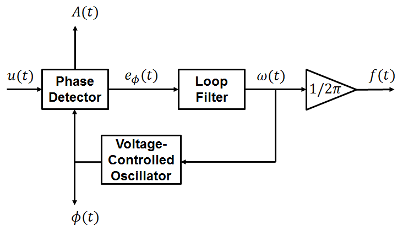# Sinusoidal Measurement (PLL, Three-Phase)

Estimate three-phase sinusoidal characteristics using a phase-locked loop

•Libraries:
Simscape / Electrical / Control / Measurements

## Description

The Sinusoidal Measurement (PLL, Three-Phase) block estimates the frequency characteristics of a balanced three-phase sinusoidal signal. The block uses a standard phase-locked loop (PLL) strategy to estimate the frequency and phase angle of the input signal. It also outputs the magnitude of the input signal.

Use this block in control applications when the frequency, phase angle, or magnitude are required and cannot be measured directly. To estimate the frequency characteristics of a non-three-phase or unbalanced sinusoidal signal, use the Sinusoidal Measurement (PLL) block instead.

### Equations

The phase-locked loop generates a sinusoid that approximates the input signal u(t) with the form:

`$y\left(t\right)=A\left(t\right)\mathrm{sin}\left({\varphi }_{0}+\int 2\pi f\left(t\right)dt\right),$`

where:

• y is the estimate of the input signal.

• A is the amplitude of the input signal.

• ϕ0 is the initial phase angle of the input signal.

Because the input signal is assumed to be balanced, the block calculates the amplitude directly from the instantaneous amplitude of the three phases. The estimated phase angle ϕ is the angle of this generated sinusoid:

`$\varphi \left(t\right)={\varphi }_{0}+\int 2\pi f\left(t\right)dt,$`

where f if the frequency of the sinusoid, and ϕ0 is the initial phase angle.

This diagram shows the overall structure of the phase-locked loop.In the diagram:

• The phase detector produces an error signal relative to the phase difference eϕ between the input sinusoid u and the synthesized sinusoid y. It also outputs the amplitude A.

• The loop filter provides an estimate of the input angular frequency ω by filtering out the high-frequency components of the phase difference. The block also outputs the converted frequency f in Hz.

• The voltage-controlled oscillator integrates the angular speed to produce the phase estimate ϕ which it sends to the Phase Detector for comparison.

## Ports

### Input

expand all

Three-phase input signal.

Data Types: `single` | `double`

### Output

expand all

Estimated frequency of the input signal, in Hz.

Data Types: `single` | `double`

Estimated phase angle of the first phase of the input signal, in rad.

Data Types: `single` | `double`

Magnitude of the input signal.

Data Types: `single` | `double`

## Parameters

expand all

Proportional gain for the loop filter. Increase this value to increase the rate at which steady-state error is eliminated in the phase angle. This value also determines the aggressiveness of the PLL in tracking and locking to the phase angle.

Integral gain for the loop filter. This determines the aggressiveness of the PLL in tracking and locking to the phase. Increase this value to reduce and eliminate steady-state error in the phase angle.

Initial estimate of the input frequency.

Initial estimate of the phase angle.

Time between consecutive block executions. During execution, the block produces outputs and, if appropriate, updates its internal state. For more information, see What Is Sample Time? and Specify Sample Time.

For inherited discrete-time operation, set this parameter to `-1`. For discrete-time operation, set this parameter to a positive integer. For continuous-time operation, set this parameter to `0`.

If this block is in a masked subsystem or a variant subsystem that supports switching between continuous operation and discrete operation, promote this parameter to ensure correct switching between the continuous and discrete implementations of the block. For more information, see Promote Block Parameters on a Mask.

## Version History

Introduced in R2017b